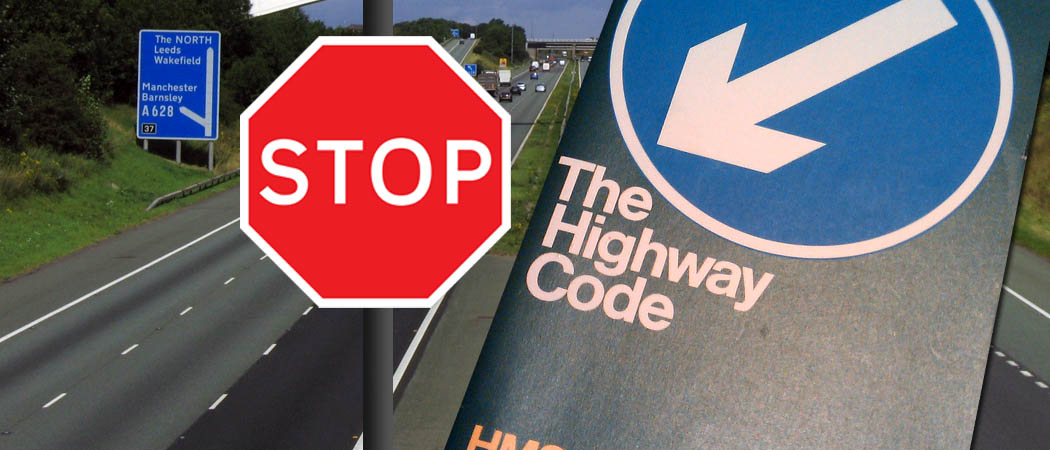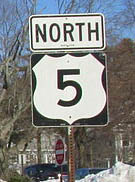# Stopping distances in the Highway Code are wrong

An easy equation to remember for your driving theory test, with dangerous consequencesTo pass your driving theory test in the UK, you need to know how far it will take you to stop if you brakes at a particular speed. But the numbers given in the Highway Code are based on inaccurate calculations that exist only because they formed an easy formula for stopping distances when we thought in feet instead of metres. Simple mechanics shows that the Highway Code systematically underestimates how long it takes to stop. At the end we propose an easy, safer equation for stopping distances.

The stopping distances you need to learn for your driving theory test are given in the Highway Code as:

Speed Stopping distance
20mph 6 + 6 = 12m
30mph 9 + 14 = 23m
40mph 12 + 24 = 36m
Speed Stopping distance
50mph 15 + 38 = 53m
60mph 18 + 55 = 73m
70mph 21 + 75 = 96m

Your stopping distance is given by thinking distance + braking distance. These numbers disguise a fascinating fact that you can only see if you write the stopping distances in feet:## The mathematical formula for stopping distances

The Highway Code gives the stopping distance in feet for a given speed, $v$, in miles per hour, as $$\text{stopping distance} = \underbrace{v}_{\text{thinking}} + \underbrace{\frac{v^2}{20}}_{\text{braking}}.$$This contains two remarkable facts:

1. Your thinking distance in feet = your speed in miles per hour
2. Your braking distance = $v^2/20$, and doesn’t depend on your vehicle’s weight.

This is remarkably coincidental considering the wildly different units: how does the maths check out?

Assuming constant speed, the distance travelled, $s$, at a speed $v$ in some time $t$ is given by $s = vt$. But this only works if we’re using the same units on both sides, so let’s write everything in feet and seconds.

There are 5280 feet in a mile, and 3600 seconds in an hour, so $$v \frac{\text{miles}}{\text{hour}} = v \times \frac{5280}{3600} \, \frac{\text{feet}}{\text{second}} \approx v \times 1.47 \, \frac{\text{feet}}{\text{second}}.$$ So in feet and seconds on both sides,$$s = v \times 1.47 \times t.$$In order for your thinking distance $s$ to equal your speed $v$, your thinking time $t$ then must equal to $1/1.47$ = 0.68 seconds. Is this fair?

By ‘thinking time’, we are really talking about reaction time: seeing there’s a problem, deciding to brake, moving your foot onto the brake pedal, and finally pushing it. The value of 0.68 seconds seems a little small for all of that, even for a fully aware driver. In fact, studies show that this time is on average considerably higher. The US uses 1.5s, and the Canadians use 2.5s.

Conclusion: the value of 0.68 seconds appears to have been chosen solely for the reason that it makes the thinking distance in feet the same as the speed in mph. Time for the Highway Code to change. Two good options:

• 2 seconds: the thinking distance in yards would equal the speed in mph.
• 2.23 seconds: the thinking distance in metres would equal the speed in mph. Perfect for a country which never quite finished metrication and is used to miles and metres.

## Your stopping distance = $v^2/20$ and doesn’t depend on your weight

The maths of this is a lovely A-level mechanics exercise. Consider the forces acting on the car when you brake. The braking force is only friction, which is proportional to the reaction force of the car from the ground:Since the reaction force $R$ equals the car’s weight $mg$, your braking force due to friction is $\mu m g$, where $\mu$ is the coefficient of friction.

By Newton’s second law, $-\mu m g = m a$, so your acceleration is therefore $-\mu g$. It’s independent of mass! So for cars and lorries, braking is all in the coefficient $\mu$. So what value are the Highway Code assuming?

Consider braking as a simple SUVAT problem: initial speed $v$, final speed 0, acceleration $-\mu g$, distance $s$. Rearranging everyone’s favourite SUVAT equation gives you $$s = \frac{v^2}{2\mu g}.$$The Highway Code equation ($s = v^2/20$) is only true for $s$ in feet and $v$ in mph. In order to compare with the SUVAT result, we need to use consistent units. If we want everything in feet and seconds, 1ft/s = 0.68mph, so we have to multiply by $0.68^2$ first: $$s = \frac{v^2 \times 0.68^2}{20} = \frac{v^2}{2\mu g}.$$Rearranging, remembering that $g$ = 32ft/s², not 10, we get $$\mu = 0.68.$$Is this good? The coefficient of friction depends on many things. On a dry day, $\mu = 0.68$ is a fair value for driving on tarmac (asphalt): most authorities use 0.7. But on a wet day, when there’s more than 0.2mm of rain on the road, $\mu$ can be as low as 0.4. And if the tread on your tyres is completely worn down, on a very wet day, $\mu$ could be even as low as 0.1.

The Highway Code states these are ‘typical stopping distances’. In the UK, it rains, on average, on 43% of days: we can hardly say that a friction coefficient for a dry road is ‘typical’. So we should reduce $\mu$. Given a bit of flexibility, then, can we pick a value for $\mu$ which is more sympathetic to metres?Walk 1m/s down here, and you’ll be going down Route 5 at Root 5 mph

If we want to use $s=v^2/2\mu g$ with $v$ in mph, we need to convert mph to m/s. 1m/s = 2.237mph, which is remarkably (and completely coincidentally) close to $\sqrt{5}$mph = 2.236mph. Therefore to convert $v^2$ in (mph)² to (m/s)², we must divide by 5:$$s = \frac{v^2/5}{2\mu g} = \frac{v^2}{100\mu}.$$Picking $\mu = 0.5$, which is in between the typical wet and dry values, we get the wonderfully neat equation that only requires knowing your square numbers up to 7:$$s = 2\left(\frac{v}{10}\right)^2.$$Conclusion: use $\mu = 0.5$.

## A safer, easier equation for stopping distances

Given the high number of variables in determining stopping distances, and the simplicity of the model, it is fair to pick values of the friction coefficent and reaction time which make the calculation easier, given that you want people to remember their stopping distances. But these numbers must still be realistic. The Highway Code, however, has historically picked values that are not realistic, and worse, continue to give estimates that are far too small. By converting their estimates into metres, all calculation ease has been lost and we are left with dangerous and forgettable data.

We suggest then, for $v$ in mph, an equation for stopping distance in metres which is both more accurate and easier to calculate:$$s = v + 2\left(\frac{v}{10}\right)^2.$$How does that stack up versus the old stopping distances? (Some common distances for scale.)With our new formula, the braking distance is mostly unchanged but the increased thinking distance makes a big impact, and rightly so. For safety and convenience, it’s time for the Highway Code to convert.

[Pictures: Banner composite: Motorway Wikimedia Commons user Klaus with K, CC-BY-SA 3.0; Highway Code Flickr user Paul Downey, CC-BY 2.0. Route 5: Wikimedia Commons user John Phelan, CC-BY-SA 3.0. Tube train: Wikimedia Commons Unisouth, CC-BY-SA 3.0. Stop sign public domain. Car: public domain. Other images: Chalkdust.]Adam is an assistant professor at Durham University, where he investigates weird, non-Newtonian fluids. If he’s not talking about the maths of chocolate fountains he is probably thinking about fonts, helping Professor Dirichlet answer your personal problems, and/or listening to BBC Radio 2.Matthew is a postdoctoral researcher at University College London. He hasn’t had time to play Klax since the noughties, but he’s pretty sure that Coke is it!